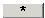# Matrix Multiplication CalGraph will multiply one matrix by another one.

Enter the first matrix on the left and the second matrix on the right.

Now click on the.

Your answer appears at the bottom middle of the window.

Multiplying matrices is picky. The number of columns in the matrix on the left must equal the number of rows in the matrix on the right. If not, the multiplication is undefined.

Warning:  the order you multiply two matrices makes a difference. Usually AB is not the same as BA.

If you want to multiply 3 matrices, enter the first two, multiply them and useto move the answer to the left side. Now enter the third matrix on the right side and click.